@liuhui0803 2017-04-01T07:39:22.000000Z 字数 6340 阅读 2480

# 使用Emoji（而非数学知识）进行深度学习

机器学习 深度学习 Emoji TensorFlow 购物

Instacart的目标是帮助客户从数百个零售商合作伙伴处订购上百万种产品。我们“购物顾问”团队中成千上万的成员必须能准确地在数千个店铺快速找到客户想要购买的商品。因此必须想方设法让这一过程尽可能快速。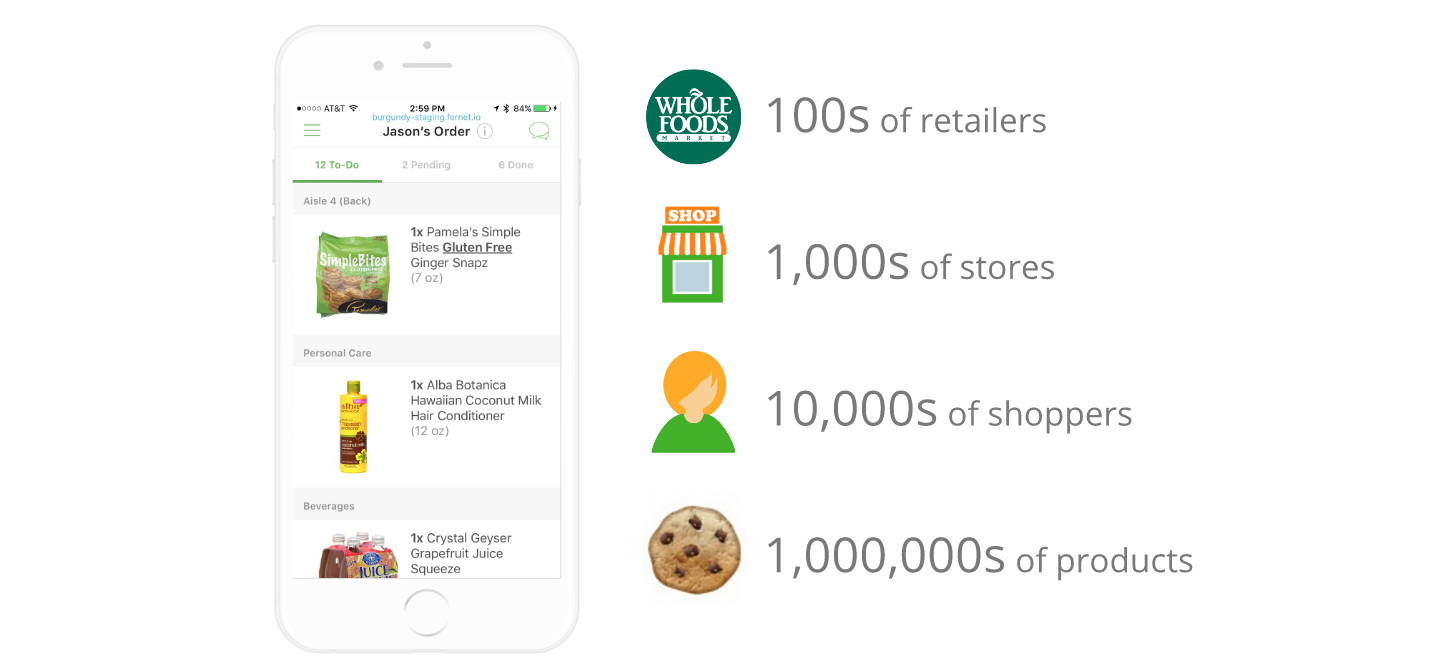## 深度学习技术“加持”的购物体验

### 深度学习入门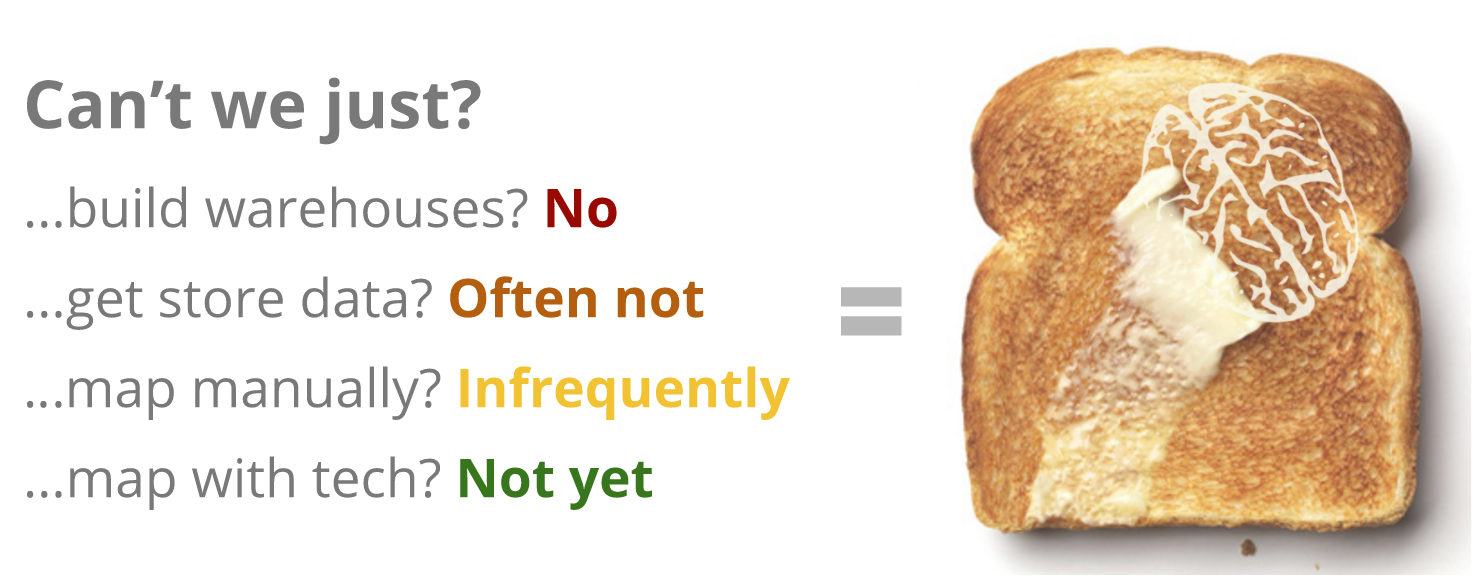### 进行过的测试

• Control（红色）：按照部门随机排序，同部门内商品按字母顺序排序；
• Human（绿色）：使用店铺陈列布局按照店铺走道排序，同走道内商品按字母顺序排序；
• TSP（青色）：使用跨部门商品挑选平均时间构建的“巡回推销员”解决方案，同部门内商品按字母顺序排序；
• Deep（紫色）：最终的深度学习架构，直接对每批中的不同商品进行排序。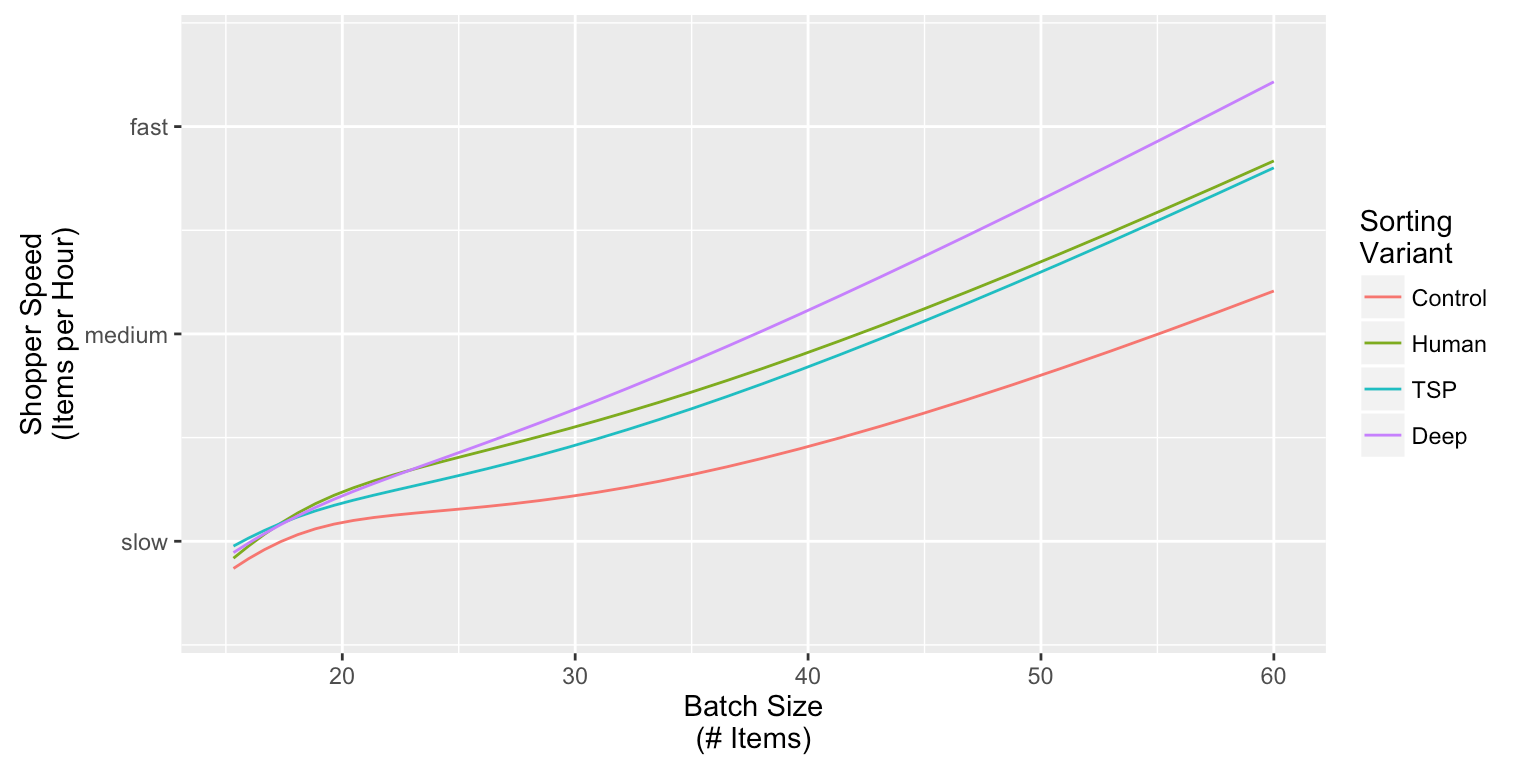Control排序方式表现最差（意料之中）。TSP和Human的表现好很多，但从统计结果来看两者没有太大差异。

## 通过Keras进行清单排序

### 问题定义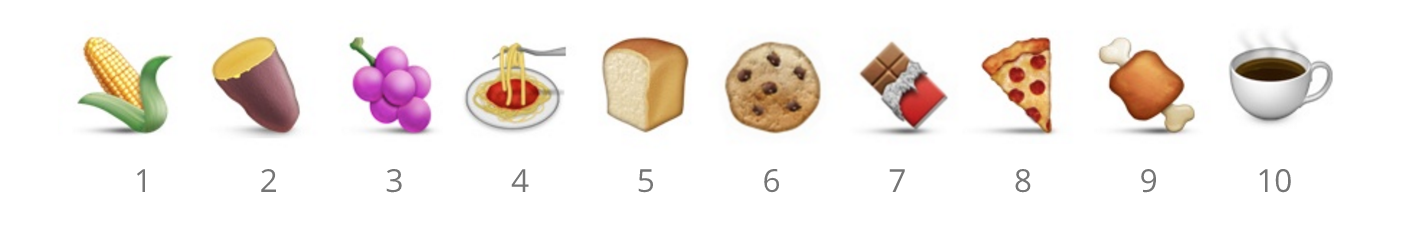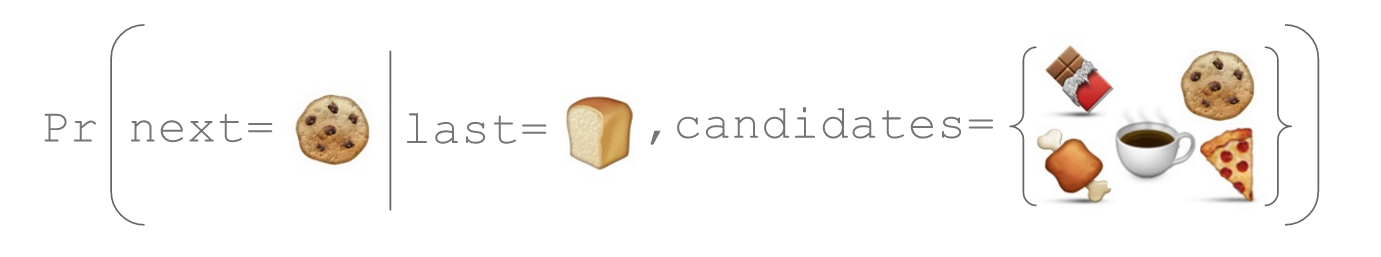### 初始架构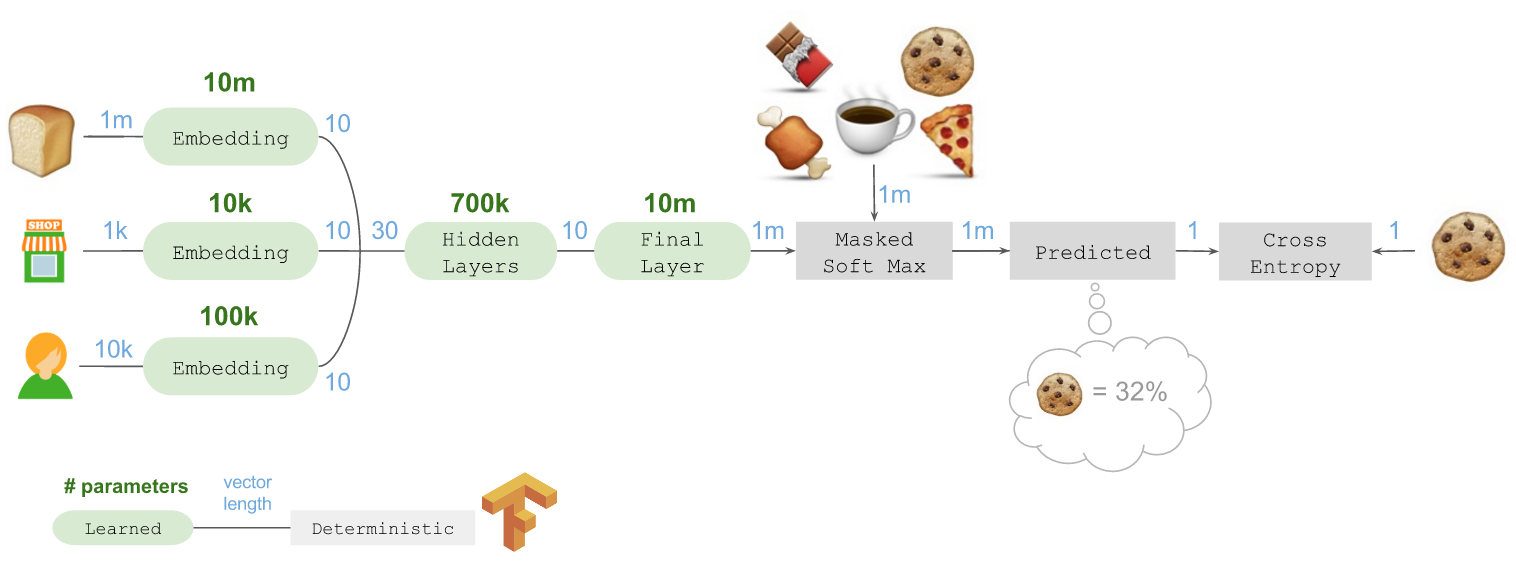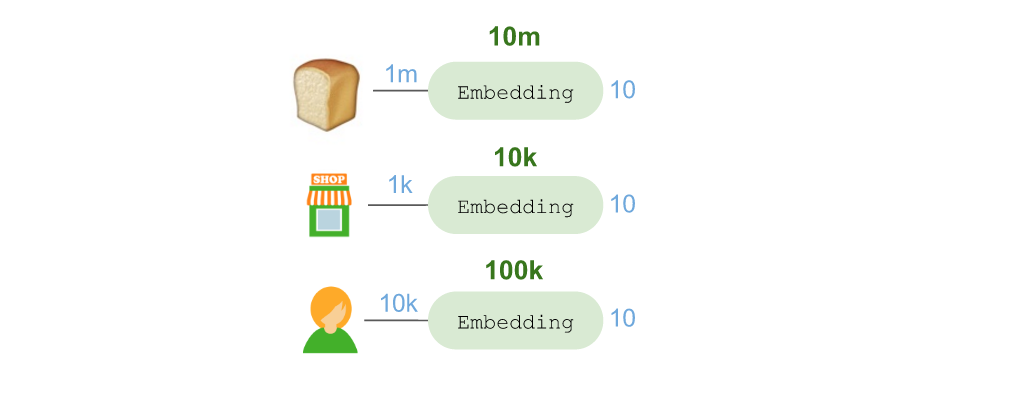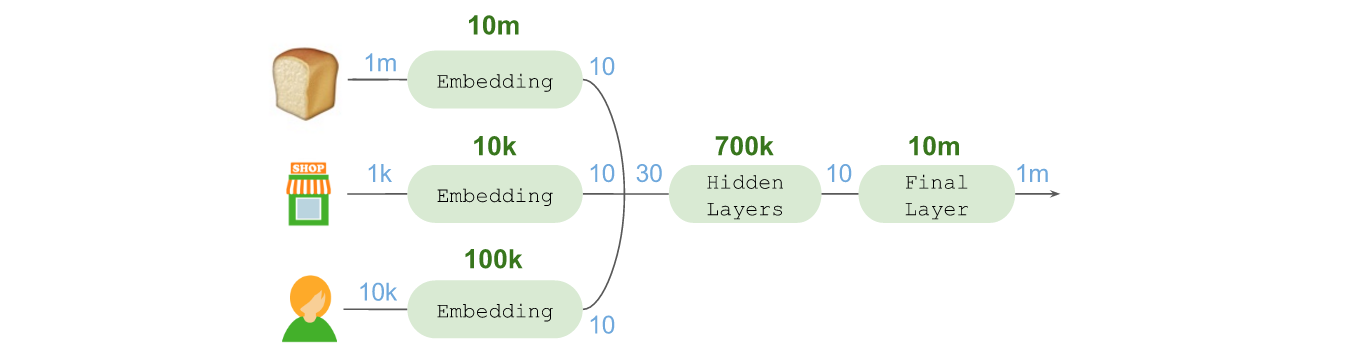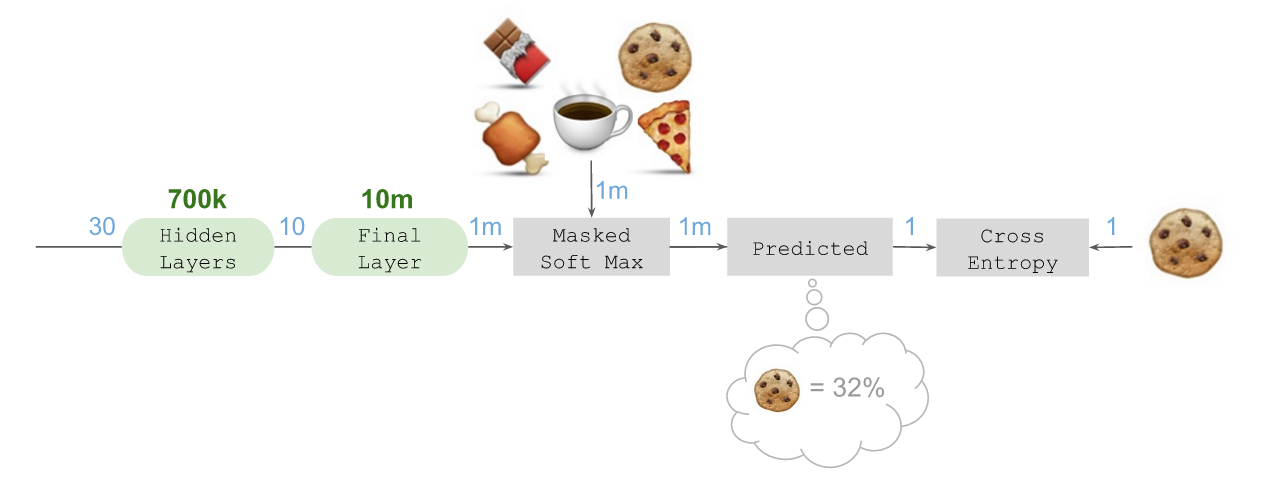### Keras代码

from keras.models import Modelfrom keras.layers.core import Dense, Reshape, Lambdafrom keras.layers import Input, Embedding, mergefrom keras import backend as K# Number of product IDs availableN_products = 1000000N_stores = 1000N_shoppers = 10000# Integer IDs representing 1-hot encodingsprior_in = Input(shape=(1,))store_in = Input(shape=(1,))shopper_in = Input(shape=(1,))# Dense N-hot encoding for candidate productscandidates_in = Input(shape=(N_products,))# Embeddingsprior = Embedding(N_products, 10)(prior_in)store = Embedding(N_stores, 10)(store_in)shopper = Embedding(N_shoppers, 10)(shopper_in)# Reshape and merge all embeddings togetherreshape = Reshape(target_shape=(10,))combined = merge([reshape(prior), reshape(store), reshape(shopper)],                 mode='concat')# Hidden layershidden_1 = Dense(1024, activation='relu')(combined)hidden_2 = Dense(512, activation='relu')(hidden_1)hidden_3 = Dense(256, activation='relu')(hidden_2)hidden_4 = Dense(10, activation='linear')(hidden_3)# Final 'fan-out' into the space of future productsfinal = Dense(N_products, activation='linear')(hidden_4)# Ensure we do not overflow when we exponentiatefinal = Lambda(lambda x: x - K.max(x))(final)# Masked soft-max using Lambda and merge-multiplicationexponentiate = Lambda(lambda x: K.exp(x))(final)masked = merge([exponentiate, candidates_in], mode='mul')predicted = Lambda(lambda x: x / K.sum(x))(masked)# Compile with categorical crossentropy and adammdl = Model(input=[prior_in, store_in, shopper_in, candidates_in],            output=predicted)mdl.compile(loss='categorical_crossentropy',             optimizer='adam',            metrics=['accuracy'])

initial_architecture.py代码托管于❤ GitHub

### 检测和局限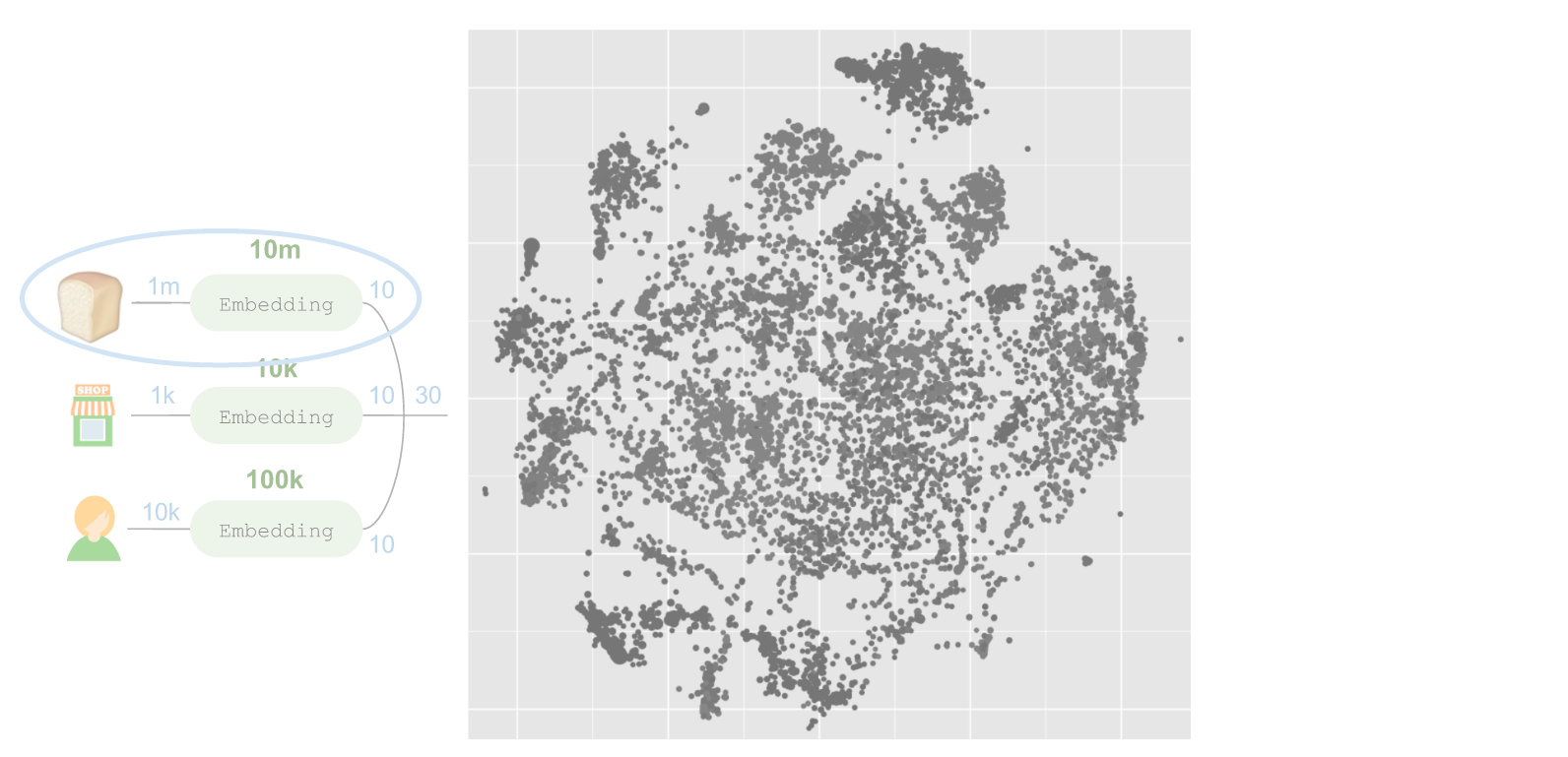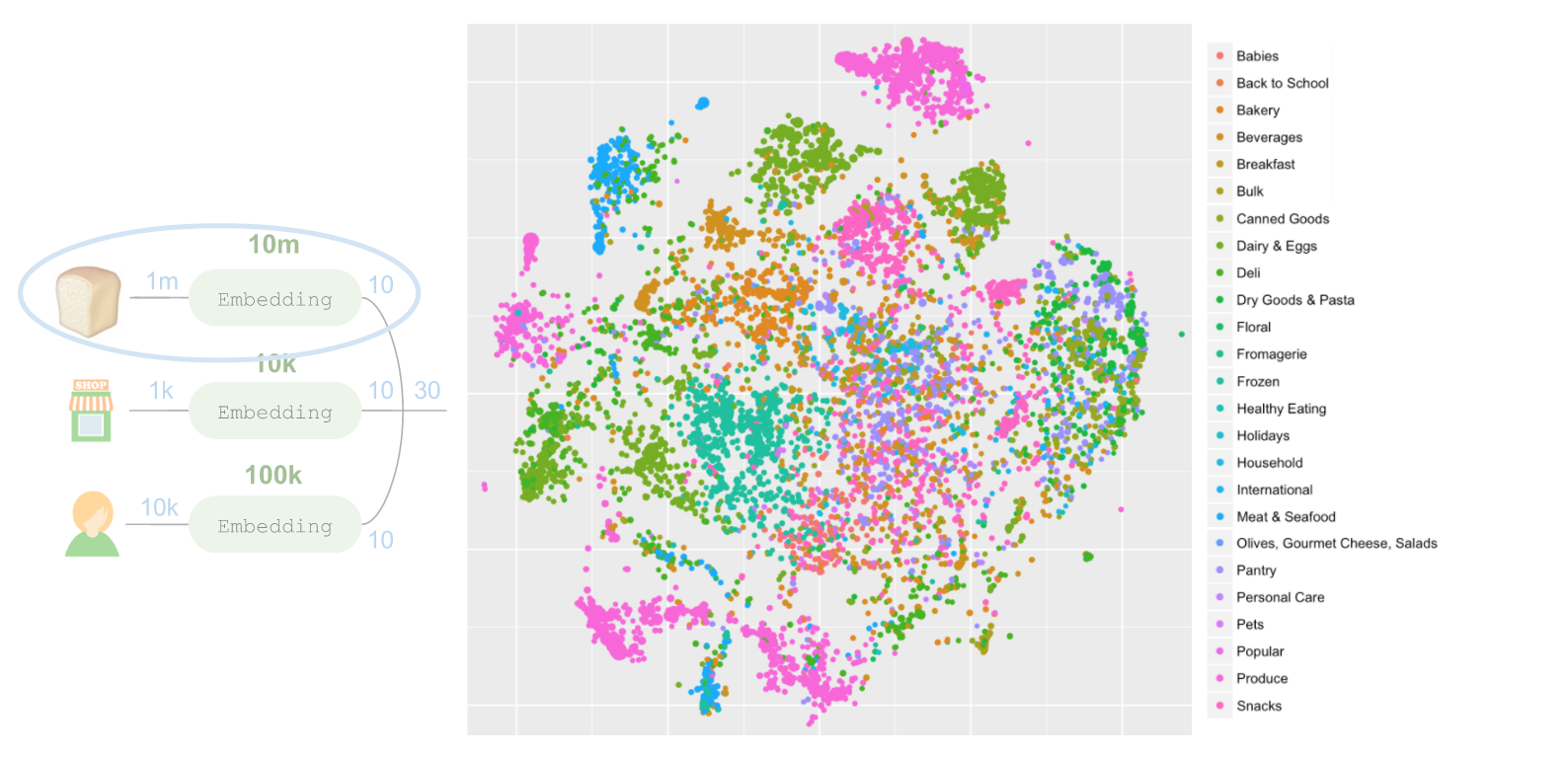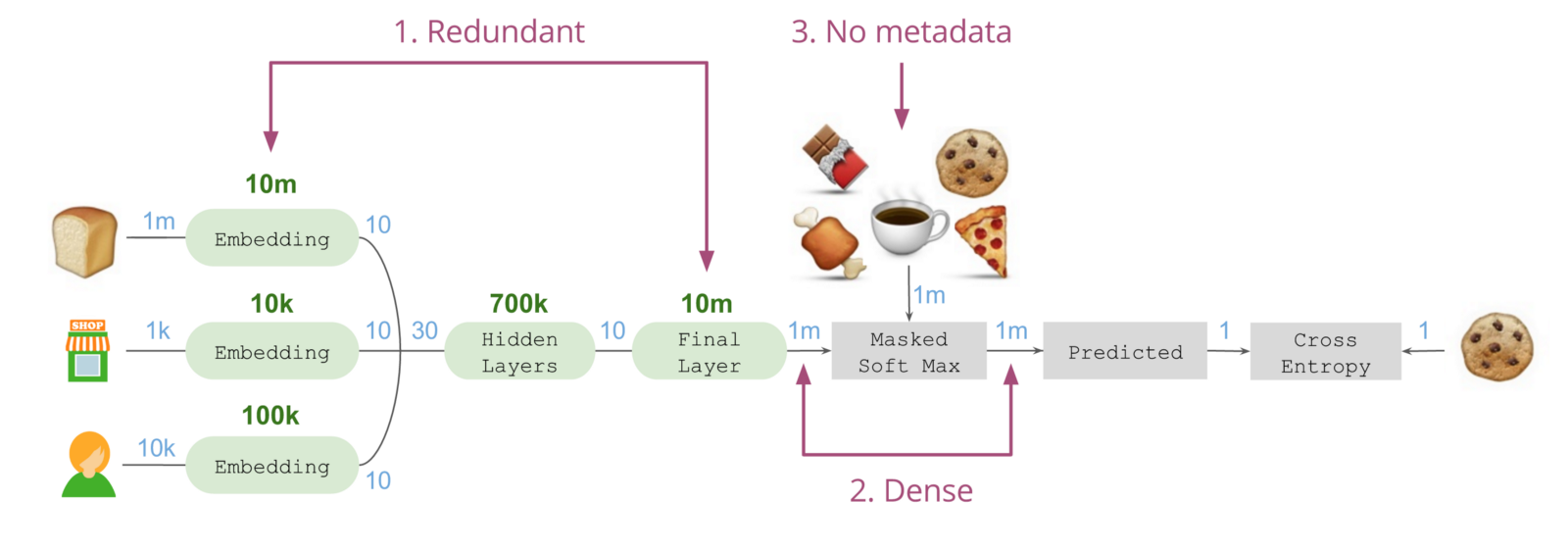### 最终架构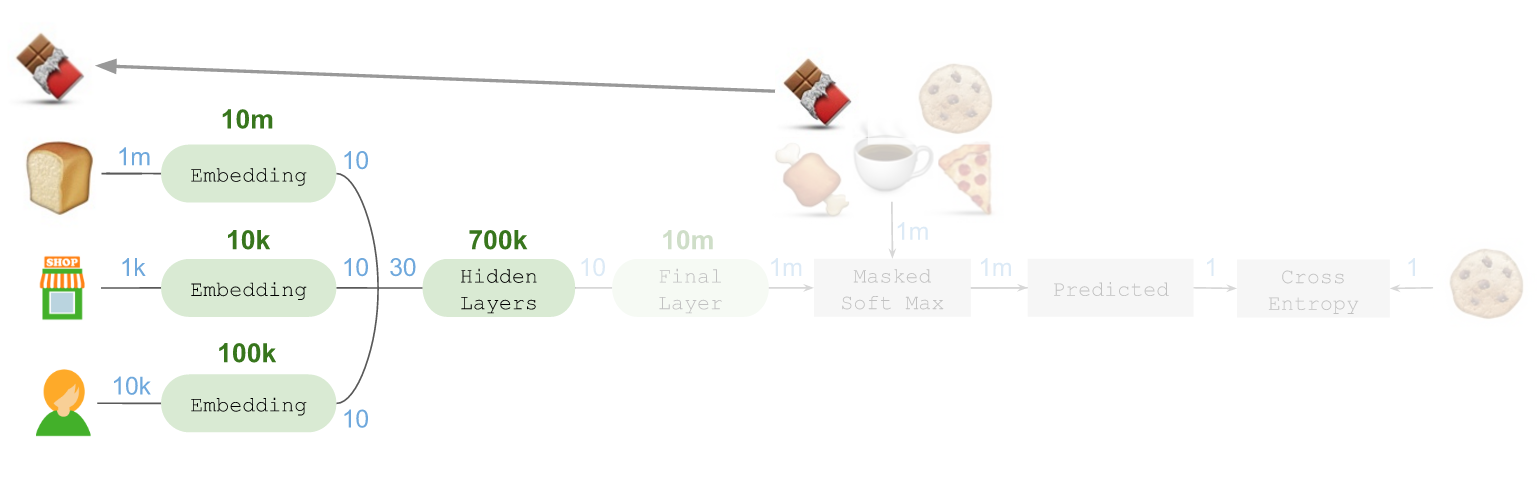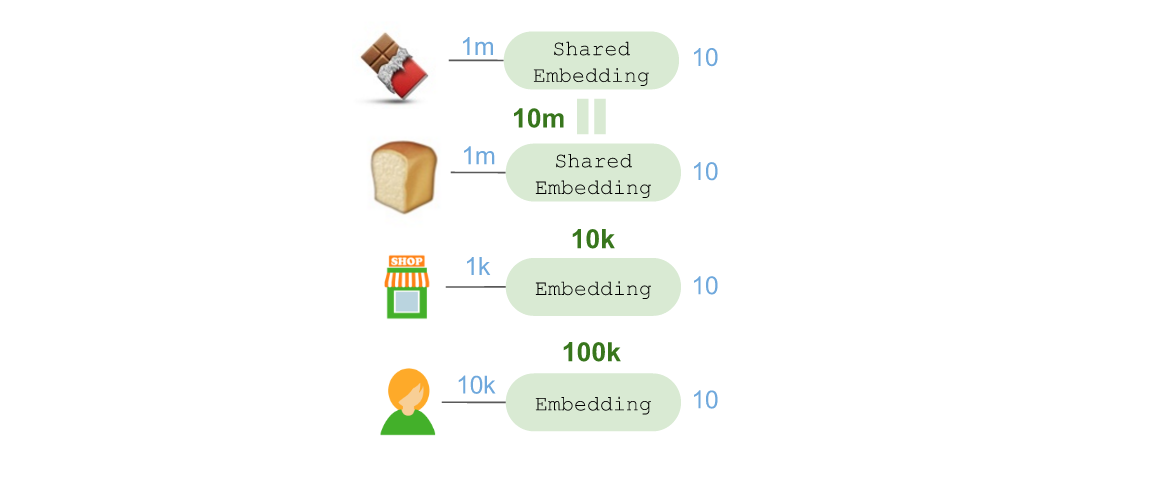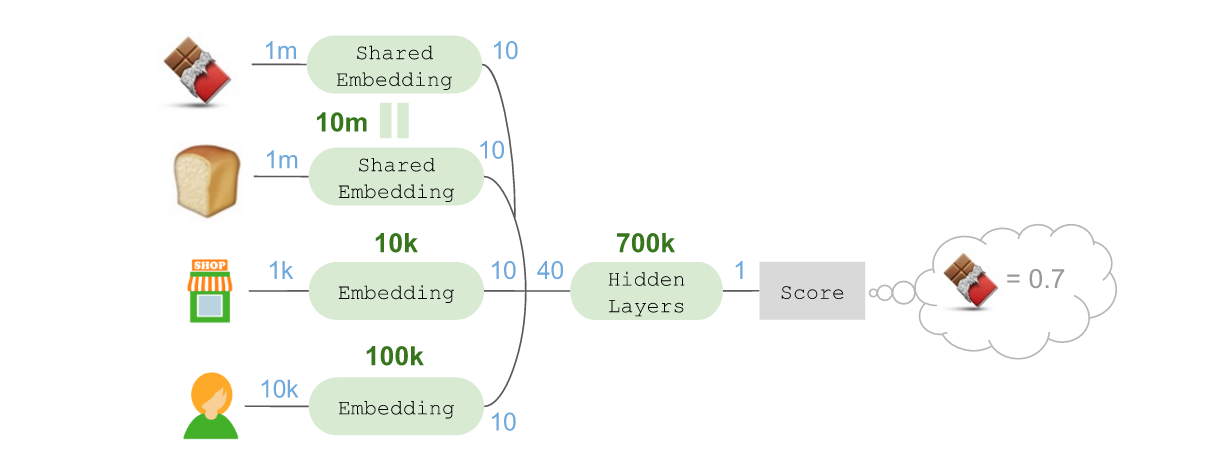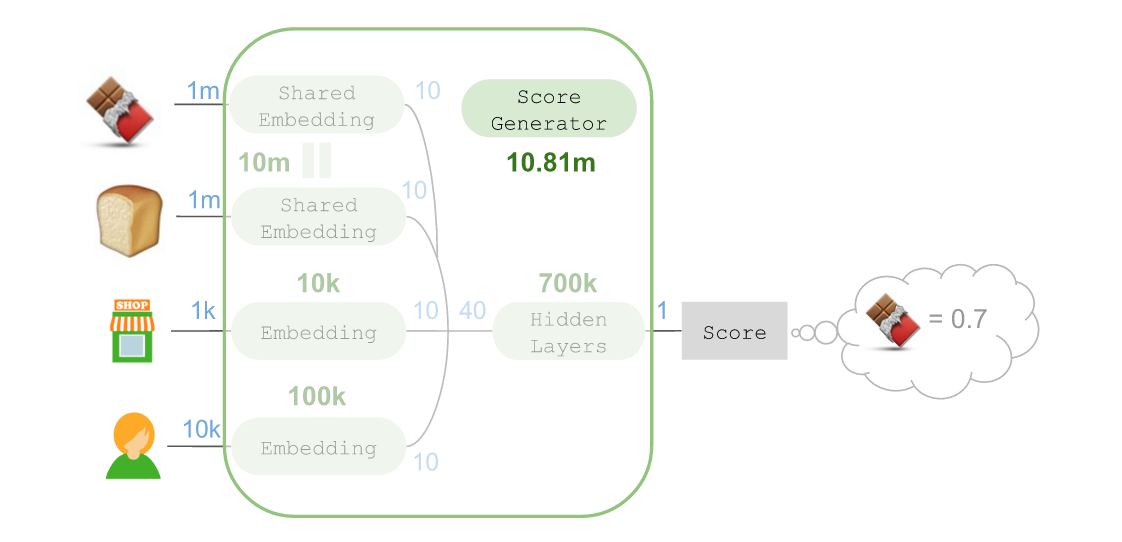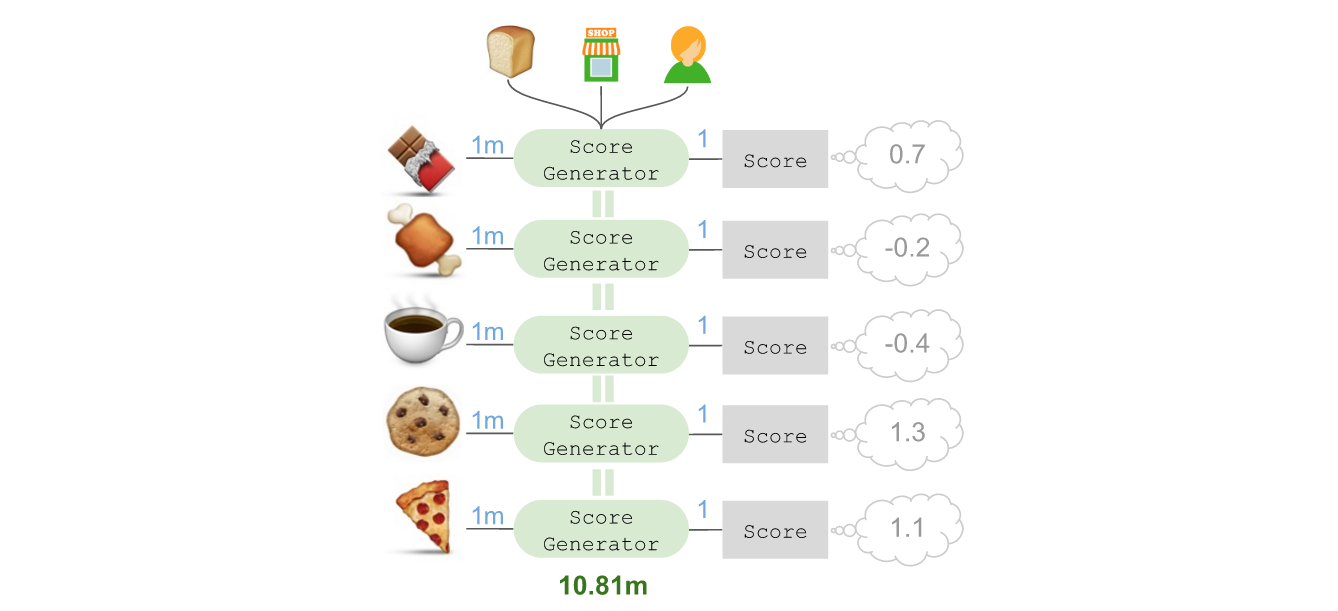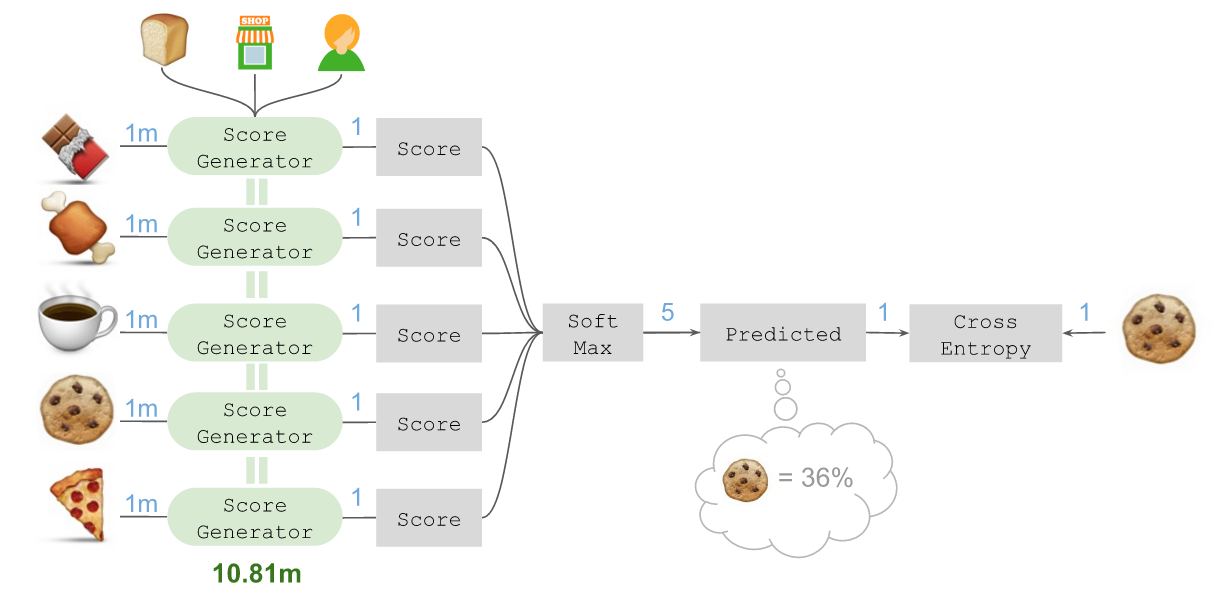## 最终结果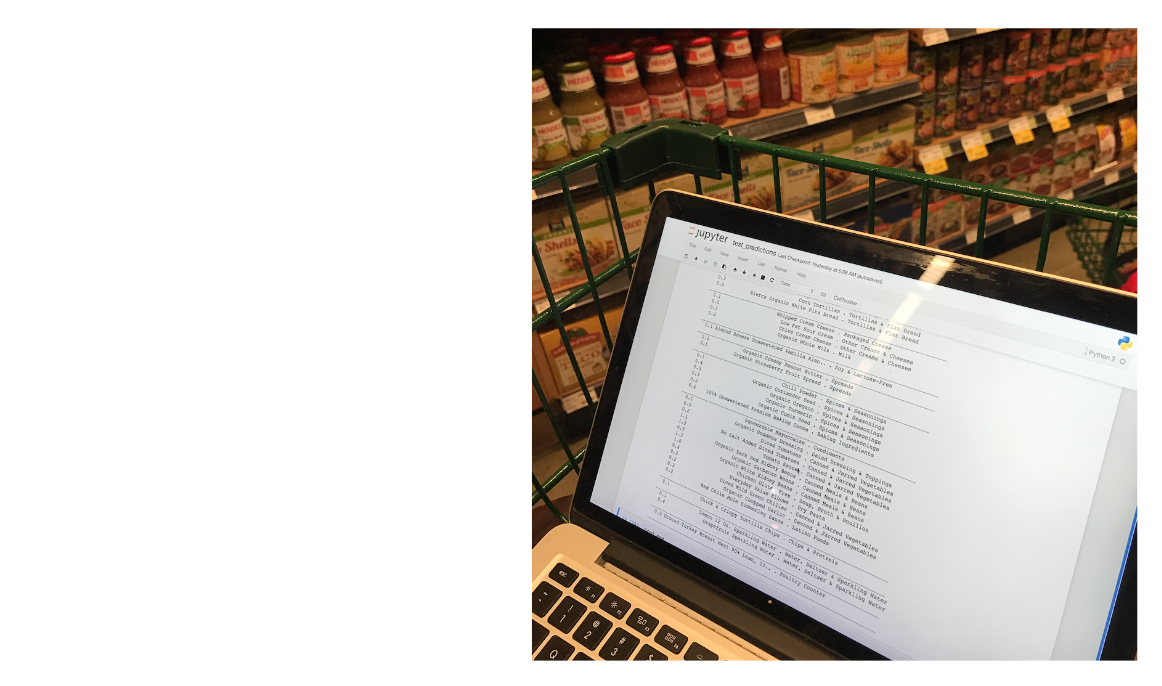• 私有
• 公开
• 删除